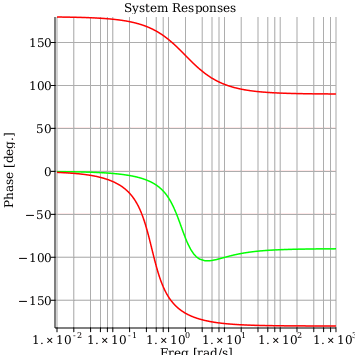Julia - Maple Help

Fractals[EscapeTime]

 Julia
 Julia fractal generatorCalling Sequence Julia( n, zbl, zur, zc ) Julia( n, zbl, zur, zc, opts )Parameters

 n - positive integer; specifies the dimensions of the square Array output zbl - complex(realcons); the bottom left corner of a box in the complex plane zur - complex(realcons); the upper right corner of a box in the complex plane zc - complex(realcons); the point in the complex plane which determines the particular Julia set opts - (optional) keyword options of the form opt=value where opt and value are described belowOptions

 • output : keyword option of the form output=value where value is a name or list of names denoting the returned Array image(s). The accepted names are layer1, layer2, color, or raw. The default value is color.
 • iterationlimit : keyword option of the form iterationlimit=value where value is a positive integer specifying the number of iterations the formula is applied. The default value is 25.
 • cutoff : keyword option of the form cutoff=value where value is positive and of type realcons. The iterative process is stopped for each complex input point when the absolute value exceeds the cutoff value. The default value is 100.0.
 • container : An n-by-n-by-2 Array with datatype=float and order=Fortran_order used in-place to store the raw data.Description

 • The Julia command generates Array images which provide a visualization of a Julia set. The entries of the image are shaded according to the behavior of complex input points under a particular iterative rational map characterized by zc a given complex parameter value. For each complex input point z a sequence of points z[i] are computed until abs(z[i]) exceeds the cutoff value (ie. escapes).

${z}_{i}={z}_{i-1}^{2}+\mathrm{zc}$

 • For each entry point in the designated complex box the iterative process is repeated until either the iteration limit or the cutoff value is exceeded.
 • The 2-D grayscale Array image returned by supplying the option output=layer1 contains data denoting the number of iterations required for each entry to escape. The grayscale image returned by supplying the option output=layer2 contains the absolute values of the final values for entries which escape. For either layer the real data is scaled to 0.0 .. 1.0 before being returned as an image.
 • The 3-D color Array image returned by supplying the option output=color contains data where the three layers corresponding to red, green, and blue have been computed using the raw escape data.
 • The 3-D Array returned by supplying the option output=raw contains the unscaled data of layer1 and layer2. This Array can be used to generate a customized color image using the Colorize command.Examples

 > $\mathrm{with}\left(\mathrm{Fractals}:-\mathrm{EscapeTime}\right)$
 $\left[{\mathrm{BurningShip}}{,}{\mathrm{Colorize}}{,}{\mathrm{HSVColorize}}{,}{\mathrm{Julia}}{,}{\mathrm{LColorize}}{,}{\mathrm{Lyapunov}}{,}{\mathrm{Mandelbrot}}{,}{\mathrm{Newton}}\right]$ (5.1)
 > $\mathrm{with}\left(\mathrm{ImageTools}\right):$
 > $\mathrm{bl},\mathrm{ur},c≔-2.0-1.5I,2.0+1.5I,-0.8+0.156I:$
 > $M≔\mathrm{Julia}\left(500,\mathrm{bl},\mathrm{ur},c\right)$
 ${{\mathrm{_rtable}}}_{{36893628828936000804}}$ (5.2)
 > $\mathrm{Embed}\left(M\right)$> $\mathrm{Embed}\left(\mathrm{Julia}\left(500,\mathrm{bl},\mathrm{ur},c,\mathrm{output}=\mathrm{layer1}\right)\right)$> $\mathrm{Embed}\left(\mathrm{Julia}\left(500,-2.0-1.5I,2.0+1.5I,1-\frac{1+\mathrm{sqrt}\left(5\right)}{2}-0.7I,\mathrm{iterationlimit}=250\right)\right)$> $\mathrm{Embed}\left(\left[\mathrm{Julia}\left(200,\mathrm{bl},\mathrm{ur},c,\mathrm{iterationlimit}=10,\mathrm{output}=\mathrm{layer1}\right),\mathrm{Julia}\left(200,\mathrm{bl},\mathrm{ur},c,\mathrm{iterationlimit}=25,\mathrm{output}=\mathrm{layer1}\right),\mathrm{Julia}\left(200,\mathrm{bl},\mathrm{ur},c,\mathrm{iterationlimit}=125,\mathrm{output}=\mathrm{layer1}\right)\right]\right)$> $\mathrm{bl},\mathrm{ur},c≔-2.0-1.5I,2.0+1.5I,-0.8+0.156I:$
 > $\mathrm{Embed}\left(\mathrm{Julia}\left(500,\mathrm{bl},\mathrm{ur},c,\mathrm{output}=\mathrm{layer2}\right)\right)$> $\mathrm{Embed}\left(\left[\mathrm{Julia}\left(200,\mathrm{bl},\mathrm{ur},c,\mathrm{cutoff}=4,\mathrm{output}=\mathrm{layer2}\right),\mathrm{Julia}\left(200,\mathrm{bl},\mathrm{ur},c,\mathrm{cutoff}=100,\mathrm{output}=\mathrm{layer2}\right),\mathrm{Julia}\left(200,\mathrm{bl},\mathrm{ur},c,\mathrm{cutoff}=10000,\mathrm{output}=\mathrm{layer2}\right)\right]\right)$> $R≔\mathrm{Array}\left(1..500,1..500,1..2,\mathrm{datatype}=\mathrm{float}\left[8\right]\right):$
 > $\mathrm{Julia}\left(500,0.-0.2I,1.5+1.0I,1-\frac{1+\mathrm{sqrt}\left(5\right)}{2}-0.7I,\mathrm{iterationlimit}=25,\mathrm{output}=\mathrm{raw},\mathrm{container}=R\right):$
 > $\mathrm{Embed}\left(\left[\mathrm{FitIntensity}\left(R\left[..,..,1\right]\right),\mathrm{FitIntensity}\left(R\left[..,..,2\right]\right)\right]\right)$> $P≔\mathrm{Colorize}\left(500,R,\mathrm{Array}\left(\left[11,3,2\right],\mathrm{datatype}=\mathrm{integer}\left[4\right]\right),\mathrm{Array}\left(\left[0.256,0.859,0.256\right],\mathrm{datatype}=\mathrm{float}\left[8\right]\right),\mathrm{rgb}=0,\mathrm{mode}=4,\mathrm{layer}=2\right):$
 > $\mathrm{Embed}\left(P\right)$Compatibility

 • The Fractals:-EscapeTime:-Julia command was introduced in Maple 18.